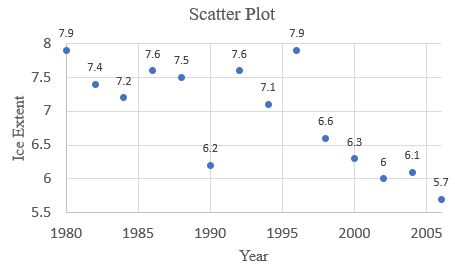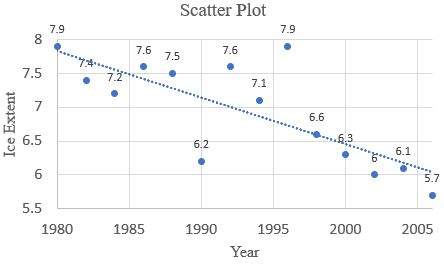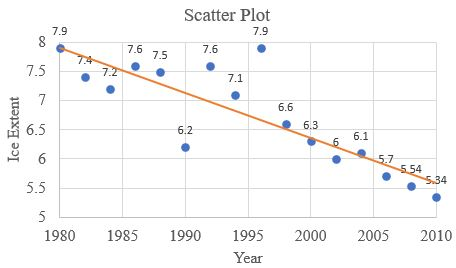# Sketch a scatter plot for the given data set.### Precalculus: Mathematics for Calcu...

6th Edition
Stewart + 5 others
Publisher: Cengage Learning
ISBN: 9780840068071### Precalculus: Mathematics for Calcu...

6th Edition
Stewart + 5 others
Publisher: Cengage Learning
ISBN: 9780840068071

#### Solutions

Chapter 1, Problem 6P

a.

To determine

## Sketch a scatter plot for the given data set.

Expert Solution

The scatter plot is### Explanation of Solution

Given: The data set is,

YearIce extent(million km2)YearIce extent(million km2)19807.919947.119827.419967.919847.219986.619867.620006.319887.520026.019906.220046.119927.620065.7

Calculation:

Using Excel to scetch a scatter plot.

• Write the data in the excel and plot using chart “scatter”.

b.

To determine

### Draw a regression line.

Expert Solution

The graph is,### Explanation of Solution

Given: The data set is,

YearIce extent(million km2)YearIce extent(million km2)19807.919947.119827.419967.919847.219986.619867.620006.319887.520026.019906.220046.119927.620065.7

Calculation:

The regression line is defined as,

y'=a+bt     .....(1)

Find the value of a and b using relation,

a=(y)(x2)(x)(xy)n(x2)(x)2b=n(xy)(x)(y)n(x2)(x)2

After simplifying the value of a and b, put into the equation (1) and get the solution.

Using Excel to scetch a scatter plot.

• Write the data in the excel and plot using chart “scatter”.

c.

To determine

### Calculate ice extent in 2010 using linear model.

Expert Solution

The ice extent in year 2010 is,

y=5.34### Explanation of Solution

Given: The data set is,

YearIce extent(million km2)YearIce extent(million km2)19807.919947.119827.419967.919847.219986.619867.620006.319887.520026.019906.220046.119927.620065.7

Calculation:

Using Excel to scetch a scatter plot.

• Write the data in the excel and plot using chart “scatter”.

### Have a homework question?

Subscribe to bartleby learn! Ask subject matter experts 30 homework questions each month. Plus, you’ll have access to millions of step-by-step textbook answers!